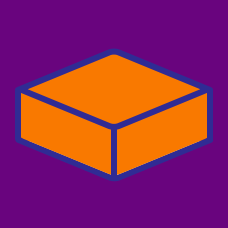Geometry

# Surface Area - Cuboid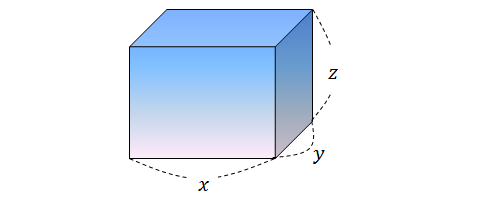In the diagram above shows a cuboid with $x=6, y=3,$ and $z=4.$ Find the surface area of the cuboid.

Note: The above figure is not drawn to scale.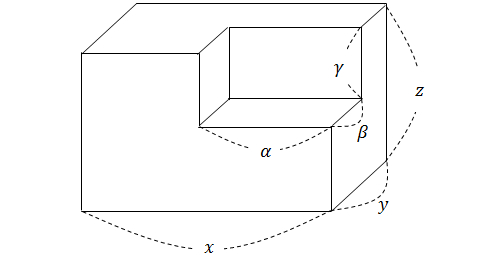The above diagram shows a large cuboid with a smaller cuboid cut off from the corner. If $\alpha=6, \beta=3,$ $\gamma=6,$ $x=11, y=6,$ and $z=12,$ what is the surface area of the remaining body?

Note: The above figure is not drawn to scale.The cuboid in the above diagram is to be tiled by square-shaped tiles on all six faces of the cuboid. If $x=10$ cm, $y=4$ cm, $z=4$ cm, and the side length of each tile is $2$ cm, how many tiles are needed?

Note: A tiling is a non-overlapping arrangement of tiles covering the entire surface area with no gaps. The above figure is not drawn to scale.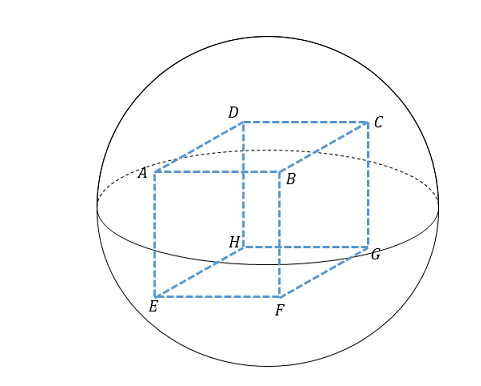What is the surface area of a cube inscribed in a sphere with radius $6,$ as shown in the above diagram?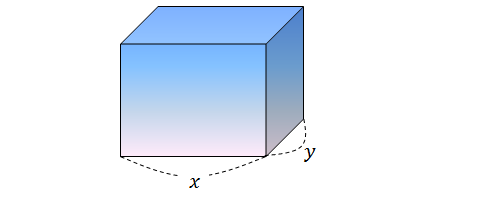The volume of the cuboid shown in the above diagram is $56.$ If $x=7$ and $y=2,$ what is the surface area of the cuboid?

Note: The above figure is not drawn to scale.

×